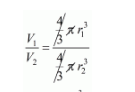# The volumes of two spheres are in the ratio 64 : 27.`
Question:

The volumes of two spheres are in the ratio 64 : 27. The ratio of their surface areas is

(a) 1 : 2

(b) 2 : 3

(c) 9 : 16

(d) 16 : 9

Solution:

Ist sphere

$V_{1}=\frac{4}{3} \pi r_{1}^{3} \ldots . .(i)$

IInd sphere

$V_{2}=\frac{4}{3} \pi r_{2}^{3} \ldots \ldots$(ii)

Divide (i) by (ii) we get,$\frac{64}{27}=\left(\frac{r_{1}}{r_{2}}\right)^{3}$

$\frac{r_{1}}{r_{2}}=\sqrt{\frac{64}{27}}$

$\frac{r_{1}}{r_{2}}=\frac{4}{3}$

Now, the ratio of their C.S.A

$\frac{S_{1}}{S_{2}}=\frac{4 \pi r_{1}^{2}}{4 \pi r_{2}^{2}}=\left(\frac{r_{1}}{r_{2}}\right)^{2}$

$\frac{S_{1}}{S_{2}}=\left(\frac{4}{3}\right)^{2}=\frac{16}{9}$

Hence, $S_{1}: S_{2}=16: 9$

Hence, the correct answer is choice $(d)$.